## Chemical Equilibrium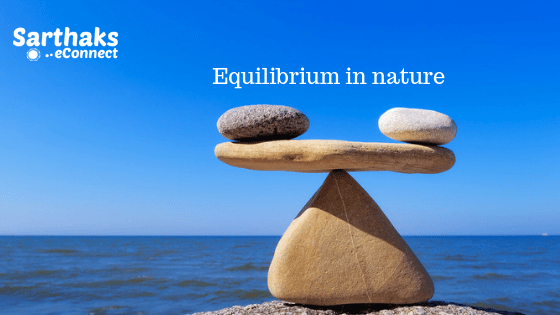• Chemical Equilibrium is a reversible reaction that occurs in a closed vessel, and a stage comes where the speed of the forward reaction equals the speed of the backward reaction
• When a chemical reaction occurs in a closed vessel at a particular temperature then first reactant concentration decreases and product concentration increases after some time there is no net reaction occurs this stage is equilibrium state.
• The concept of chemical equilibrium was developed after Berthollet (1803) found that some chemical reactions are reversible.
• For any reaction mixture to exist at equilibrium, the rates of the forward and backward (reverse) reactions are equal.

### Dynamic equilibrium:

• The ratio of reactants/products changes, but substances move between the chemicals at an equal rate, meaning there is no net change
• Many chemical reactions are reversible, and the forward and backward reactions can occur at the same time. When the rate of the forward reaction is equal to the rate of the backward reaction, call as a dynamic equilibrium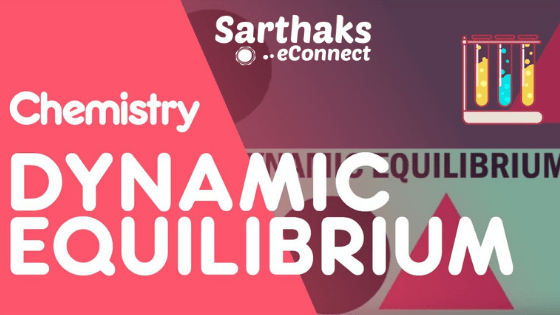• Chemical equilibrium is important in numerous biological and environmental processes.So,
• For example, equilibria involving O2 molecules and the protein hemoglobin play a crucial role in the transport and delivery of O2 from our lungs to our muscles.
• Similar equilibria involving CO molecules and hemoglobin account for the toxicity of CO.
• The mixture of reactants and products in the equilibrium state is called an equilibrium mixture.
• Under a given set of conditions if a reversible process or chemical reaction is carried out in a closed container, constancy in some observable properties like
• colour intensity, pressure, density, is observed.
• Such a state is referred to as an equilibrium state.
• The extent of a reaction in equilibrium varies with the experimental conditions such as:
•  concentrations of reactants, temperature, etc.
• Optimization of the operational conditions is very important in industry and laboratory so that equilibrium is favorable in the direction of the desired product.
• Physical and Chemical Processes:
Physical processes involve such changes, which only acts the physical properties of the substance undergoing changes but have no effect on the chemical composition and properties.
Chemical processes involve changes in chemical composition and properties. Whenever a chemical change occurs, we can say that a chemical reaction has taken place.

#### Types of Chemical Reactions in Chemical equilibrium:• Combination Reactions: In such reactions, two or more substances combine to form a single compound. e.g., 2Mg + O2→ 2MgO
• Decomposition reaction: When a compound decomposes to form two or more substances. e.g.,
PCl5   ⇔   PCl3  +   Cl2
•  Digestion of food is also a decomposition reaction.
•  Displacement Reactions: These reactions involve the displacement of one element or group by another. Hence these are redox reactions. e.g., Zn(s) + H2SO4  ⇔  ZnSO4  +  H2
•  Double Displacement or Metathesis Reactions: In these reactions, two compounds react to form two new compounds and no change in oxidation state take place, e.g., precipitation reactions, neutralization, reactions.
 Reversible Irreversible Reactions Reactions which do not proceed to competition in forward direction & also proceed in backward directions under suitable condition.e.g     N2(g)+ 3H2(g) ⇄ 2NH3 Reactions only proceed to competition in a forward direction. AgNO3 + NaCl → AgCl + NaNO3 These reactions attain equilibrium. These reactions never attain equilibrium

## Equilibrium Classification:

Physical Equilibrium:
Equilibrium set up in physical processes like the evaporation of water, melting of solids, dissolution of solutes, etc., is called physical equilibrium, e.g.,    Ice ⇔ Water   At equilibrium,  Rate of melting of ice = Rate of freezing of water.
Chemical Equilibrium

Is a reversible reaction is carried out in a closed vessel, a stage is attained where the speed of the forward reaction equals the speed of the backward reaction. It corresponds to chemical equilibrium. At equilibrium,
Rate of forward reaction = Rate of backward reaction.### Characteristics of Chemical Equilibrium:

• Equilibrium can be attained from either side
•  Equilibrium is dynamic in nature, i.e., at equilibrium reaction does not stop.
•  At equilibrium, there is no change in the concentration of various species.
•  The equilibrium state remains unaffected by the presence of a catalyst. Catalyst helps to attain the equilibrium state rapidly.
• The observable physical properties of the process become constant.

Types of Equilibrium:

1. Homogeneous Equilibrium:  In homogeneous equilibrium, the reactants and products are present in the same phase or physical state (gaseous or liquid).

2SO2 (g) + O2 (g) ⇔ 2SO3 (g)

2. Heterogeneous Equilibrium: In heterogeneous equilibrium, the reactants and products are present in two or more physical states or phases.

3Fe(s) + 4H2O (g) ⇔ Fe3O4 (s) + 4H2 (g)

## Law of Mass Action in Chemical equilibrium:

Guldberg and Waage state that the rate of a chemical reaction is directly proportional to the product of the active masses of the reacting substances. Hence For a general reaction,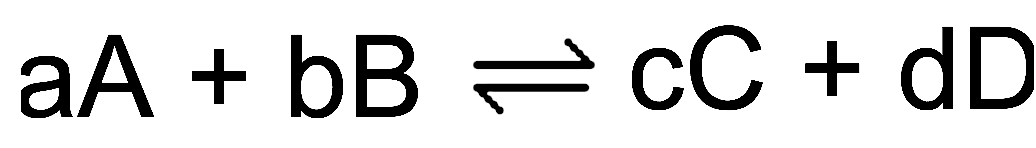Rate of forward reaction  ∝ [A]a [B]b = kf [A]a [B]b

Rate of backward reaction  ∝ [C]c [D]d = kb[C]c [D]d
Where kf & kb are rate constant.

• In heterogeneous equilibrium the active mass of pure solid and liquid are:
Rate of forward reaction= Rate of backward reaction.
kf [A]a [B]b = kb[C]c [D]d
kf/ kb = kc = [C]c [D]d/[A]a [B]b
K or Kc = Equilibrium constant

### Characteristics of  Partial pressure or Equilibrium constant :

• It has definite value for every chemical reaction at a particular temperature.
• The more is the value of Kc or Kp, the more is the extent of completion of the reaction, i.e., K < 1 indicates a lesser concentration of products than reactants. K ≥ 10 shows completion of the reaction and K ≤ 10 shows that the reaction does not proceed at all.
• When the reaction can be expressed as a sum of two other reactions, the Kc of the overall reaction is equal to the product of equilibrium constants of individual reactions.
•  The equilibrium constant is independent of initial concentrations of reactants.
•  The equilibrium constant is independent of the presence of a catalyst.
• Kc for a backward reaction is inverse of Kc for the forward reaction.
• If an equation is multiplied by n, the K becomes Kn , and if it is divided by m, the k becomes m√k.
•  In equilibrium constant expression if activities are used in places of the molar concentration, h becomes dimensionless.

## Use of Partial Pressures Instead of Concentration:

For gaseous reactions, partial pressures are conveniently used since at any fixed temperature partial pressure is directly proportional to concentration. For a general gaseous reaction.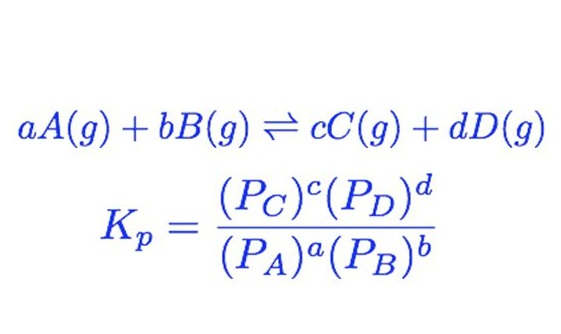Relation between Kc and Partial pressure:Where, Δng = moles of products – moles of reactants (gaseous only).

Relation between Equilibrium constant  and Partial pressure for different types of reactions:

 Δng 0 +ve, -ve KP or Kc KP = Kc Kp > Kc Kp < Kc

#### Units of Kp and Equilibrium constant:

• Unit of Kp = (atm) Δng
• Unit of Kc= (mol L-1) Δng

## Reaction Quotient:

• For any reversible reaction at any stage other than equilibrium.
• The ratio of the molar concentrations of the products to that of the reactants.
• Where each concentration term is raised to the power equal to the stoichiometric coefficient to the substance concerned, is the reaction quotient, QC .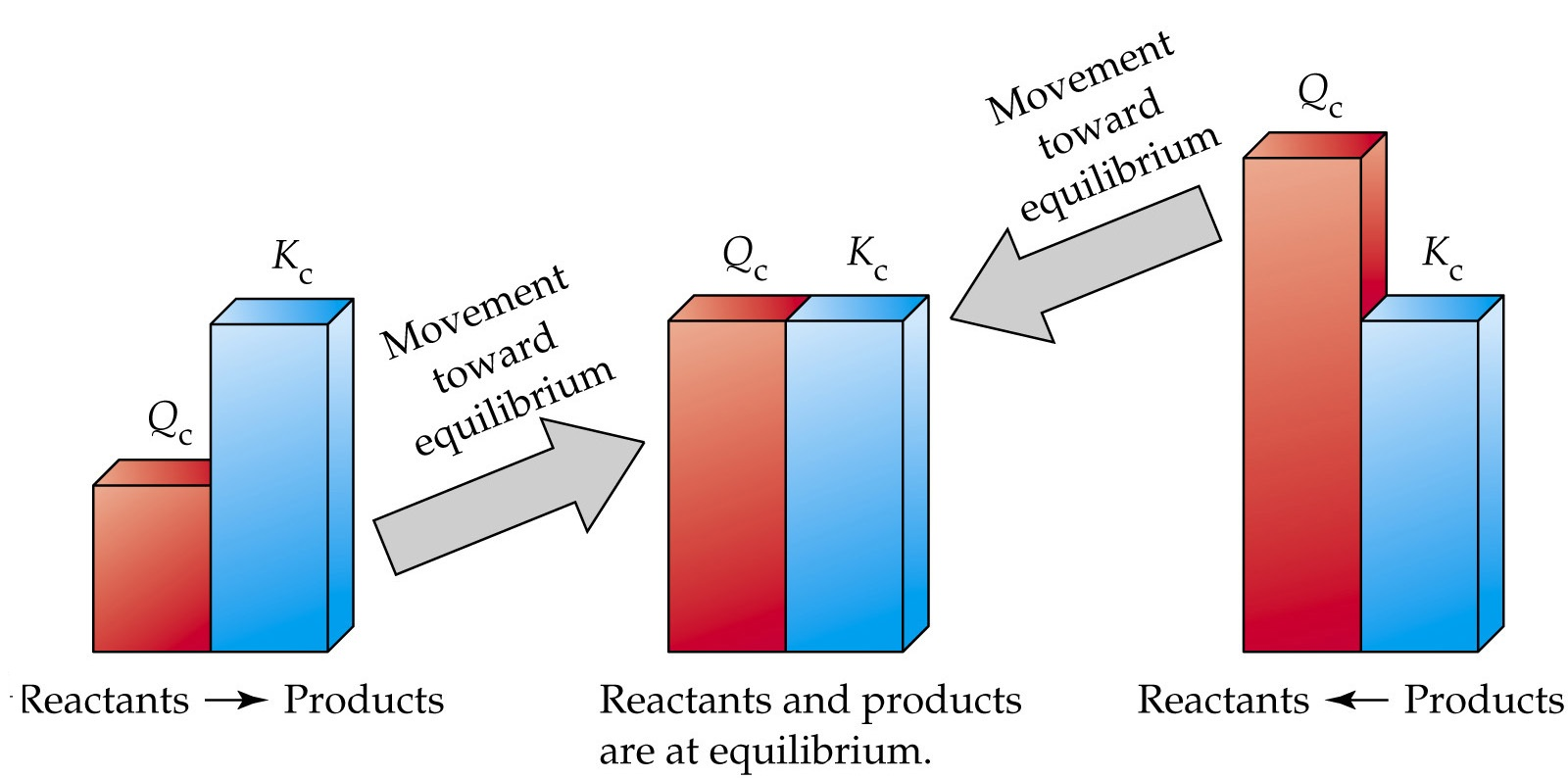For a general reaction:       aA     +     bB      ⇄    cC    +     dD

Qc  =  [C]c  [D]d / [A]a [B]b## Le – Chatelier’s Principle:

• Le – Chatelier’s principle states that if a system at equilibrium is subjected to a change in concentration, pressure or temperature. The equilibrium shifts in the direction that tends to nullify the effect of change.
• There are three main factors which affect the state of equilibrium. They are:
• Concentration, Temperature, Pressure.

### Effect of Change of Concentration:

If at equilibrium the concentration of one of the reactants is increased. The equilibrium will shift in the forward direction and vice-versa.

#### Effect of Change in Pressure:

• No effect of pressure on equilibria having same moles of reactants and products.

e.g. N2 + O2⇔ 2NO.

• When there is a change in the number of moles, the equilibrium will shift in the direction having a smaller number of moles when the pressure is increased and vice-versa,

e.g., N2 + 3H2 ⇔ 2NH3   [High p. high yield of NH3]

##### Effect of Temperature

When the process is exothermic, low temperature favors the forward reaction. Whereas a process is endothermic.  high temperature favors the formation of products.

###### Effect of Addition of Inert Gas
• Addition of inert gas at constant pressure:

If inert gas is added. It will increase the volume of the system. Therefore. The equilibrium will shift in a direction in which there is an increase in the number of moles of gases.

• Addition of inert gas at constant volume:

If keeping volume of the system constant, an inert gas is added. The relative molar concentration of the substance will not change. Hence. The equilibrium position of the reaction remains unaffected.

•  Effect of Catalyst:

The presence of catalyst does not change the position of equilibrium. Hence it simply fastens the attainment of equilibrium.

## Le-Chatelier’s Principle Applicable to Physical Equilibrium:

• Effect of pressure on solubility:

The increased pressure will increase the solubility of gas and vice-versa.

• Effect of temperature on solubility:

Some substances dissolve with the absorption of heat. So the solubility of such substances will increase with the increase of temperature and vice-versa, e.g., dissolution of NH4CI, KCI, KNO3 , etc. The dissolution of calcium acetate and calcium hydroxide is exothermic, so their solubility is lowered at a higher temperature.

• Effect of pressure on the melting point of ice:

Ice ⇔ liquid water. The ice occupy the more volume than liquid water, so increased pressure will result in       melting of ice according to Le-Chatelier’s Principle

### Favorable conditions for some chemical equilibria to get a higher yield of product:

• N2 + 3H2  ⇄  2NH3    (At high pressure, low Temperature & isolation of NH3 by liquefaction.)
• N2 + O2  ⇄   2NO   (At high Temperature & isolation of NO)
• PCl5  ⇄  PCl3 +Cl  (At low pressure, high Temperature & isolation of PCl5 & Cl2)

## Calculation of the Degree of Dissociation (α) from Density Measurements in Chemical equilibrium:

α = D – d /d (y-1)
Where,

D = theoretical vapour density
d = observed vapour density
Now,
Molecular mass = 2 x VD
∴                                                            α = Mc – Mo / Mo
Where,
Mc = calculated molecular weight.
Mo = observed molecular weight.

## Important Question & Answers in Chemical equilibrium:

1.  Find out Kc for the given reaction in equilibrium state: [SO2]= 0.6 M, [O2] = 0.82 M and [SO3] = 1.9 M ?

ANS: As per the question,

2SO2 (g) +O2 (g) ↔2SO3 (g)       (Given)

Kc = [SO3]2/ [SO2]2 [O2]

= (1.9)2 M2/(0.6)2 (0.82) M3

= 12.229 M-1

2.  For the following equilibrium, K =   6.3×1014 at 1000K
NO (g) +O3 (g) ↔NO2 (g) +O2 (g)
Both the reverse and forward reactions in the equilibrium are elementary bimolecular reactions. Calculate Kc, for the reverse reaction?

ANS:

For the reverse reaction,               Kc = 1/Kc
= 1/6.3×1014
= 1.5×10-15

3. At equilibrium, the concentrations of N2= 3.0 × 10–3M, O2 = 4.2 ×10-3M & NO= 2.8 ×10-3M in a sealed vessel at 800K. What will be Kc for the reaction N2 (g) + O2 (g) ⇄ 2NO (g)

ANS:

For the reaction equilibrium constant Kc is written as :

Kc = [NO]2 / [N2] [O2]

= (2.8 10-3 M)2 / (3.0 x 10-3 M) (4.2 x 10-3 M)

= 0.622

4. PCl5 ,PCl3 & Cl2 are at equilibrium at 500 K and having concentration 1.59 M PCl3, 1.59 MCl2 &   1.41 M PCl5. Calculate Kc for the reaction,
PCl5 ⇄ PCl3 + Cl2

ANS:

Equilibrium constant Kc for above reaction is:
PCl5 ⇄ PCl3 + Cl2

Kc = [PCl3] [Cl2] / [PCl5]

=  (1.59)2 / (1.41)

= 1.79.

1.Komal Singh September 5, 2019